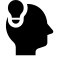# 矩阵求导的Trace Trick

## 公式推导

Posted by viewsetting on May 17, 2020

# 规则

• $d(\mathbf{X} \pm \mathbf{Y}) = d\mathbf{X} \pm d\mathbf{Y}$ ，加减运算可以直接提出来
• $d({\mathbf{X1}...\mathbf{Xi}...\mathbf{Xn}}) = \sum_{i=1}^{n} \mathbf{X1}...(d\mathbf{Xi})...\mathbf{Xn}$ 矩阵乘法的微分，注意每个分量中的矩阵顺序不能改变，这是由矩阵乘法不可交换的性质决定的。
• $d(X^\top) = (dX)^{\top}$ 转置可以提出来
• $d (tr(\mathbf{X})) = tr(d\mathbf{X})$ trace同上

•  $$d X = tr(X^{#}dX)X^{#}$为$X$$的伴随矩阵
• $d(X \circ Y) = dX\circ Y + X\circ dY$ $\circ$为矩阵的内积，即逐元素积
• $d (\sigma(X)) = \sigma^{'}(x) \circ dX; \sigma(X) = [\sigma(X_{ij})]$ 这里是将作用于标量的函数拆出，通过对应矩阵生成函数矩阵，然后将其与矩阵的微分$dX$乘起来。

• $x = tr(x)$ 标量转换
• $tr(X^\top) = tr(X)$ 转置trace一样
• $tr(A+B) = tr(A)+tr(B)$ 线性拆分
• $tr(A^\top B) = tr(B^\top A)$ 满足交换律
• $tr(A^\top (B \circ C)) = tr((A \circ B)^\top C)$，这里三个矩阵$A,B,C$的大小相同。证明略。

# 举例

### 1

$f = a^\top X b$，其中：$a \in R^{m \times 1}, b \in R^{n \times 1}, X \in R^{m \times n}$

$f$求微分： $df = da^\top Xb +a^\top dX b+a\top Xdb$ 由于$a,b$$X$无关，所以一三项消去，得到： $df = a^\top dX b$ 由于$df$为标量，所以引入trace： $df = tr(a^\top dX b)$ 通过交换律: $df = tr(ba^\top dX)$ 由： $df=tr((\frac{\partial f}{\mathbf{X}})^\top d\mathbf{X})$ 得到: $\frac{\partial f}{\mathbf{X}} = (ba^\top)^\top = ab^\top$

### 2

$f = X^\top A X$，其中：$A \in R^{m \times n}, X \in R^{m \times n}$

$f$求微分： $df = dX^\top AX +X^\top dAX+X^\top AdX$ 消去$dA$，得到： $df = dX^\top AX +X^\top AdX$ 等式右边引入trace运算,同时通过trace的线性分解性质得到: $df = tr(dX^\top AX) + tr(X^\top AdX)$ 又由于trace中转置不变性，将第一项内的矩阵乘积转置后得到$dX$项，为： $df = tr((AX)^\top dX) + tr(X^\top AdX)$ 由于trace的线性，最后将两个项合并得到： $df = tr((X^\top A+(AX)^\top) dX)$ 所以： $\frac{\partial f}{\mathbf{X}} =(X^\top (A+A^\top)^\top = (A+A^\top)X$

### 3 线性回归的最小二乘法求导